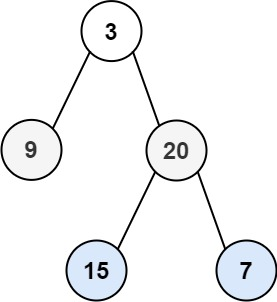# Binary Tree Zigzag Level Order Traversal

## Binary Tree Zigzag Level Order Traversal

Given the `root` of a binary tree, return the zigzag level order traversal of its nodes' values. (i.e., from left to right, then right to left for the next level and alternate between).

Example 1:```Input: root = [3,9,20,null,null,15,7]
Output: [,[20,9],[15,7]]
```

Example 2:

```Input: root = 
Output: []
```

Example 3:

```Input: root = []
Output: []
```

Constraints:

• The number of nodes in the tree is in the range `[0, 2000]`.
• `-100 <= Node.val <= 100`

### Solution

/**
* Definition for a binary tree node.
* struct TreeNode {
*     int val;
*     TreeNode *left;
*     TreeNode *right;
*     TreeNode() : val(0), left(nullptr), right(nullptr) {}
*     TreeNode(int x) : val(x), left(nullptr), right(nullptr) {}
*     TreeNode(int x, TreeNode *left, TreeNode *right) : val(x), left(left), right(right) {}
* };
*/
class Solution {
public:
vector<vector<int>> zigzagLevelOrder(TreeNode* root) {
vector<vector<int>>v;
if(root==NULL){
return v;
}
stack<int>st;
vector<int>temp;
queue<TreeNode*>q;
q.push(root);
q.push(NULL);
int i=1;
while(!q.empty()){
TreeNode* f=q.front();
q.pop();
if(f==NULL){
if(i%2==0){
while(!st.empty()){
int k=st.top();
st.pop();
temp.push_back(k);
}
}
v.push_back(temp);
temp.clear();
i++;
if(!q.empty()){
q.push(NULL);
}
}
else{
if(i%2==0){
st.push(f->val);
}
else{
temp.push_back(f->val);
}
if(f->left){
q.push(f->left);
}
if(f->right){
q.push(f->right);
}
}
}
return v;
}
};